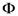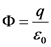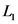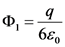# A point charge of magnitude q is at the center of a cube with sides of length L

A point charge of magnitude q is at the center of a cube with sides of length L.

A. What is the electric flux Φ through each of the six faces of the cube? Use ϵ0 for the permittivity of free space.
B. What would be the flux Φ1 through a face of the cube if its sides were of length L1? Use ϵ0 for the permittivity of free space.

Concept and reason
Electric flux is the measure of flow of the electric field through a given area.
The electric flux can be determined using the number of field lines passing through a given area.
The electric flux through a surface can be calculated using the charge enclosed in a surface.

Fundamentals
Gauss law: For a closed Gaussian surface, the electric flux is given by,

(A)
Consider a cube of side length L in which a charge of magnitude q is placed at the center.
Consider a closed Gaussian surface which is cube whose electric flux iswhich is given by,The number of field lines emerging from charge will be divided into six faces.
So, the electric flux is given by,Part A
The electric flux through each of the six faces of the cube isThe electric flux is defined as the number of field lines passing per unit area. The flux passing through any face of the cube is equal to the total flux through the cube divided by six.

(B)
Consider the sides of the cube is.
As, the electric flux depends only on the enclosed charge q, the flux through each face would be same as previous part even if the dimension of the cube is changed. Hence, the electric flux through each of the six faces of the cube whose lengthis,Part B
The electric flux through each of the six faces of the cube isAs the flux depends upon the charge inside the closed surface, the flux through each face would be same as previous part even if the dimension is changed.